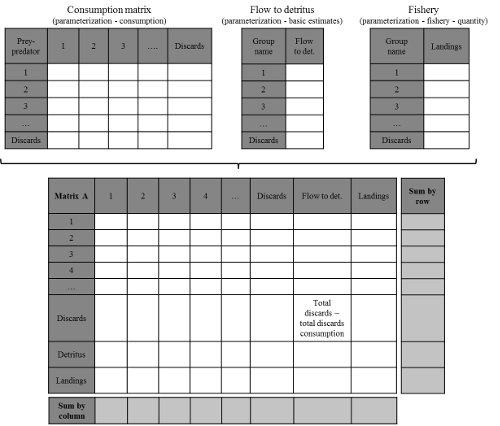# Sensitivity of Inverse Community matrix

## R-script for inverse community matrix

This script is the supplemental material of two publications on trophic network analyses. This allows to test for uncertainties of direct and indirect effects of small variation in biomass of a trophic network compartment on the rest of the ecosystem.

### Construction of the Community matrix from Ecopath modelsFigure 1: Community matrix construction from Ecopath

### The R-code for the sensitivity analysis

The code is given for data stored in a three-sheet Excel file. The original matrix Q is stored in the “net_impacts” sheet and is obtained following steps (i) to (iv) described in Appendix C. The original matrix Q minus 20% is in the “Com_Min” sheet and the original matrix Q plus 20% in the “Com_Max” sheet. They all three give the net impact of a compartment in rows on the compartment in column.

``````library(RODBC)

#Specify the number of matrix Q to simulate
nb_mat <- 5000

#Connection to the Excel file and importation of the data
db <- "" #File path
channel <- odbcConnectExcel(xls.file = db)

Mini_Mat <- sqlFetch(
channel = channel,
##Name of the Excel source sheet with the original matrix Q minus 20%
sqtable = "Com_Min",
rownames = TRUE
)
Mini_Mat <- Mini_Mat[,-1]

Maxi_Mat <- sqlFetch(
channel = channel,
##Name of the Excel source sheet with the original matrix Q plus 20%
sqtable = "Com_Max",
rownames = TRUE
)
Maxi_Mat <- Maxi_Mat[,-1]

#Creation of two empty matrices to store the 5000 simulated Q and MTI matrices
nb_comp <- nrow(Mini_Mat)
strenght <- matrix(0,nrow=nb_comp,ncol=nb_comp*nb_mat)
strenght_adj <- matrix(0,nrow=nb_comp,ncol=nb_comp*nb_mat)

#Function to invert a matrix Q following Dambacher et al. (2002)
#With the following equation, the CM matrix must be invertible
#No compartment being only an input can be included in the model. Effect of an input may be regarded after the matrix has been inverted
#Compartment being only an output is allowed since the diagonal has been set to -1 for auto-regulation
make.adjoint2 <- function(CM,status=FALSE) {
adj2 <- (-1)* solve(CM)*det(- (CM))
adj2
}
#The resulting MTI matrix returns the effect of a positive perturbation in a compartment in rows on a compartment in column
#Simulation of the 5000 Q matrices by drawing q ij values from independent uniform distributions. Minimums and maximums for the distributions were defined according to Mini_Mat and Maxi_Mat

for(t in 1:nb_mat){
col_j <- (nb_comp*t)-nb_comp
for(i in 1:nb_comp){
for(j in 1:nb_comp){
strenght[i,j+col_j] <- runif(1,Mini_Mat[i,j],Maxi_Mat[i,j])
}
}
#Simulation of the 5000 MTI matrices using the make.adjoint2 function to invert the 5000 Q matrices
begin <- (nb_comp*t)-nb_comp + 1
end <- nb_comp*t
strenght_adj[,begin:end] <- make.adjoint2(strenght[,begin:end])
}

#Transformation of quantitative MTI values, with 1 for positive integers, 0 for nulls and -1 for negative integers
effect <- strenght_adj
for(i in 1:nb_comp){
for(j in 1:(nb_comp*nb_mat)){
if(strenght_adj[i,j]>0){effect[i,j] <- 1
} else {
if(strenght_adj[i,j]==0){effect[i,j] <- 0
}else{
effect[i,j] <- (-1)
}
}
}
}

#Counting for each intersection the number of positive, negative and null MTI values and storage into three summary matrices ( effect_po , effect_neg and effect_nul , respectively)
effect_pos <- matrix(0,ncol = nb_comp, nrow=nb_comp)
effect_neg <- matrix(0,ncol = nb_comp, nrow=nb_comp)
effect_nul <- matrix(0,ncol = nb_comp, nrow=nb_comp)

for(t in 1:nb_mat){
for(i in 1:nb_comp){
for(j in 1:nb_comp){
if(effect[i,(j+((t*nb_comp)-nb_comp))]==1){effect_pos[i,j] <- effect_pos[i,j]+1
} else {
if(effect[i,(j+((t*nb_comp)-nb_comp))]==(-1)){effect_neg[i,j] <- effect_neg[i,j]+1
} else {
effect_nul[i,j] <- effect_nul[i,j]+1
}
}
}
}
}

#For each intersection, sum of the three summary matrices should be equal to the number of simulations (here 5000). This test must return TRUE.
(sum(effect_neg + effect_pos + effect_nul - matrix(nb_mat,ncol=nb_comp,nrow=nb_comp)) == 0)

#Importation of the original Q matrix
Reference <- sqlFetch(
channel = channel,
#Name of the Excel source sheet with the original matrix Q
sqtable = "net_impacts",
rownames = TRUE
)

Reference <- as.matrix(Reference[,-1])
#Inversion of the original Q matrix resulting in the original MTI matrix
Reference <- make.adjoint2(Reference)
#Transformation of the original MTI matrix into three matrices. For a given intersection, if the original MTI value is positive (negative, null), it should be specified in the effect_pos_ref ( effect_pos_neg , effect_pos_nul ) matrix by putting the number of simulations (here 5000) at the corresponding intersection
effect_pos_ref <- matrix(0,nrow=nb_comp,ncol=nb_comp)
effect_neg_ref <- matrix(0,nrow=nb_comp,ncol=nb_comp)
effect_nul_ref <- matrix(0,nrow=nb_comp,ncol=nb_comp)

for(i in 1:nb_comp){
for(j in 1:nb_comp){
if(Reference[i,j] > 0){effect_pos_ref[i,j] <- nb_mat}
if(Reference[i,j] < 0){effect_neg_ref[i,j] <- nb_mat}
if(Reference[i,j] == 0){effect_nul_ref[i,j] <- nb_mat}
}
}

#For each intersection, calculation of the proportion of simulations with the same sign as in the original MTI matrix
#Proportion is the final matrix used in the paper to assess sensitivity of the MTIs to 20% uncertainty in the input data.
proportion <- matrix(0,ncol = nb_comp, nrow=nb_comp)

for(i in 1:nb_comp){
for(j in 1:nb_comp){
if(effect_pos_ref[i,j] == nb_mat){proportion[i,j] <-(effect_pos[i,j]/nb_mat)*100}
if(effect_neg_ref[i,j] == nb_mat){proportion[i,j] <-(effect_neg[i,j]/nb_mat)*100}
if(effect_nul_ref[i,j] == nb_mat){proportion[i,j] <-(effect_nul[i,j]/nb_mat)*100}
}
}``````

###### Citation:

For attribution, please cite this work as:
``` Rochette Sébastien. (2015, Jul. 23). "Sensitivity of Inverse Community matrix". Retrieved from https://statnmap.com/2015-07-23-sensitivity-of-inverse-community-matrix/. ```

BibTex citation:
``````@misc{Roche2015Sensi,
author = {Rochette Sébastien},
title = {Sensitivity of Inverse Community matrix},
url = {https://statnmap.com/2015-07-23-sensitivity-of-inverse-community-matrix/},
year = {2015}
}
``````
Comments
comments powered by Disqus